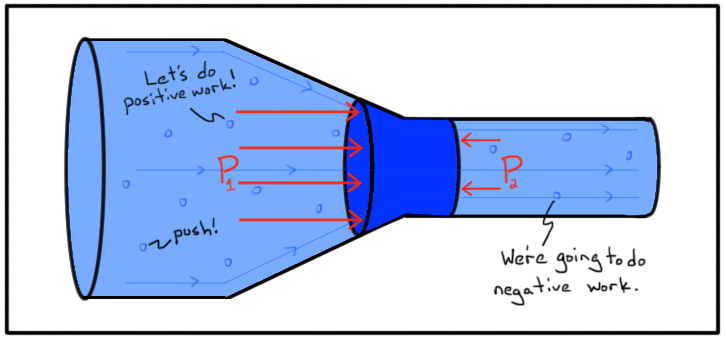## Saturday, September 2, 2017

### Bernoulli's Principle

Published by Daniel Bernoulli in 1738, Bernoulli's Principle is an important principle in fluid mechanics, and has many applications in the real world, including generating lift for aerofoils.

Bernoulli's Principle states that the faster a fluid moves, the less pressure or potential energy the fluid has. This principle is based on the conservation of energy: at all times, the energy in a system has to remain constant. Thus, if a fluid starts to move faster, it will gain kinetic energy and dynamic pressure (the pressure exerted by a moving object) and lose potential energy and static pressure (the pressure exerted by a static object). This ensures that the amount of energy in the fluid remains constant.

Bernoulli's Equation

Bernoulli's Principle is a principle that is obtained from Bernoulli's equation. This equation relates the pressure, speed and height of a liquid at two different points in a steady flow. The equation is as follows:
Here, all the values marked with 1 apply to the first point in the flow, while those marked with 2 indicate the second point in the flow. P refers to the pressure, V to the velocity, g to gravitational acceleration and h to the height. The rho symbol refers to the density of the liquid.

This equation states that the sum of the pressure, kinetic energy density and potential energy density of a liquid at any two points in a laminar flow are equal, which is basically the conservation of energy for liquids. Bear in mind that this equation assumes a great many things, such as smooth flow, an in-compressible liquid, lack of any outside outside forces, among others. However, it works.

Bernoulli's equation relates the energies of a liquid flowing from one height to another. Bernoulli's principle is a simplification of this. It relates the pressure and speed of a liquid at two different points in a horizontal line.

Please do bear in mind that this equation is valid only for in-compressible fluids flowing at low speeds. There are more advanced equations for compressible fluids flowing at high speeds.

(Above): Bernoulli's equation.

Bernoulli's Principle

As mentioned earlier, Bernoulli's principle relates the pressure and speed of a liquid at two different points in a horizontal line. This means that, from the above equation, there will be no difference in potential energy density, as there is not difference in height. So those two terms cancel each other out.

Now we can say that the sum of the pressure and kinetic energy density of the fluid at point 1 is equal to the sum of the same at point 2. Thus, for constant pressure and kinetic energy density at point 1, if there is an increase in the speed of the liquid at point 2, it must be accompanied by a drop in the pressure at point 2. This point is elaborated on below.In a system like the one pictured above, water is flowing from the wide side to the narrow side. For the liquid to flow at the same rate, it must speed up at the narrow section. But if speed increases, so does kinetic energy, and this energy has to come from somewhere. Since the water is flowing toward the right, it can only be assumed that it is being pushed along by the slower water to the left, indicating that the pressure at point 1, in the slower bit of water, is more than that at point 2, in the faster stream. And this is the essence of the principle: the faster a liquid goes, the less pressure it exerts.

(Above): Water flowing from a wide area to a narrow area of a pipe.

Applications

There are several applications of Bernoulli's Principle in real life, but I will focus on the aeronautical one here.

An aerofoil shape moving through a fluid causes the fluid underneath it to flow slower than the fluid on top. From the principle, we know that the faster a fluid moves, the lesser the pressure it exerts. Thus, the fluid on top exerts less pressure than the fluid at the bottom. This causes the underlying fluid to exert pressure on the underside of the wing, lifting it up. This force which it exerts is known as lift.

Thus, if we know the speeds of the fluids on top and bottom, we can calculate the force of lift quite accurately using Bernoulli's equations. The equations, however, do not explain why the fluids flow faster over the top than the bottom.